• 在word中输入latex公式
多人点赞
2022-07-16 09:15:52

CSDN 叶庭云https://yetingyun.blog.csdn.net/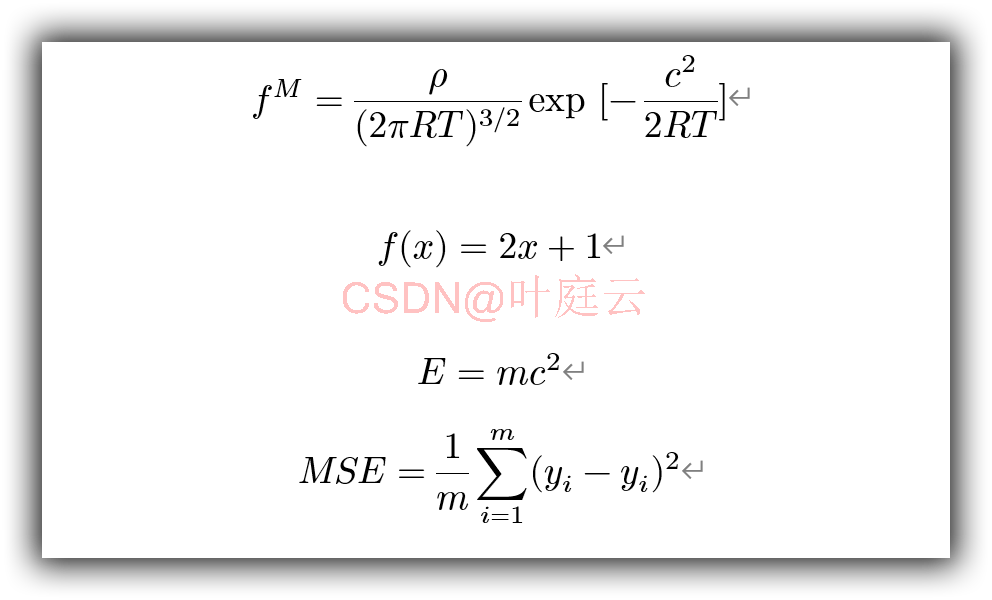其实 Word2016 以上自带的公式编辑器挺好用的。它除了可以通过鼠标点击插入公式以外，也完全支持像 LaTex 一样通过键盘输入公式。而且还可以使得写出的公式像 LaTex 里一样漂亮，具体操作步骤如下：

• 按快捷键 Alt= 进入公式输入模式（会出现公式编辑框）；
• 用像 LaTex 一样的语法键入公式，比如上标可以用 ^ ；
• 输入完成后选择公式—转换，点击全部—专业（A）渲染一遍即可得到漂亮的 LaTex 公式（设置过的话，直接 Enter 键回车即可）。

如下所示：Word 默认的公式字体是：CambrLaTex
更多相关内容
• 下面就简单介绍下WordLaTex数学公式输入功能，这里不讲LaTex的相关语法，默认已经了解。我使用Word版本是Word for mac 2019，与Windows版功能上应该没有差别，UI界面可能有些不同。 \qquad先将光标移动到需要插入...

\qquad Word 是我们常用的办公软件，用于编写文档，写论文等。具有十分强大的宏、域、加载项，大部分人只是用到了其中的一小部分功能。下面就简单介绍下Word的LaTex数学公式输入功能，这里不讲LaTex的相关语法，默认已经了解。我使用Word版本是Word for mac 2019，与Windows版功能上应该没有差别，UI界面可能有些不同。
\qquad 先将光标移动到需要插入公式的地方，可使用Ctrl + =快捷键插入公式，然后就出现的公式输入框：\qquad Word支持的公式输入模式有：Unicode、LaTex、文本。\qquad 然后即可在公式输入框输入LaTex数公式：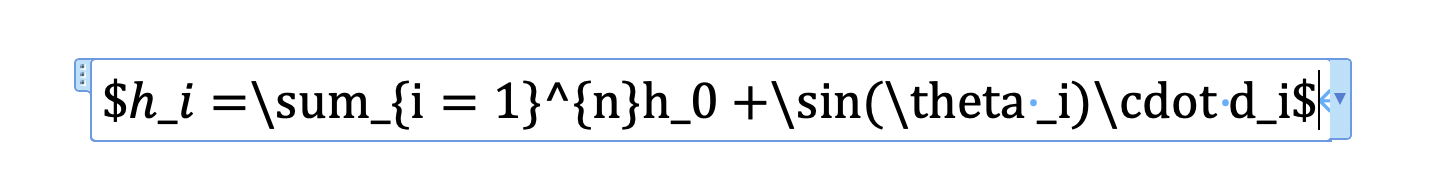\qquad 点击回车键，即可生成输入的公式：\qquad 对于复杂的公式可以直接使用LaTex输入，尤其是在写文章或写书的时候，可能涉及到大量复杂的公式，其中不乏矩阵、级数等形式复杂的公式。相较于Word自带的公式编辑器，LaTex公式输入的效率要高出很多。但Word 并不支持全功能的LaTex，部分表达式暂不支持。

展开全文latex
• 在Word中使用Latex输入公式

# Word中使用Latex输入公式

office word 2019版本中自带LaTex编辑器，以office word 2019为例：

1. 将光标移到需要插入文献的位置，在word工具栏中选择：插入 → 公式；快捷键：“Alt”+"="1. 在word工具栏中选择：公式 → 转换 → LaTex；word刚开始时默认的为Unicode，在更改为LaTex之后就会保存设置为LaTex，下次插入公式时就不需要再次更改了。2. 在word文档中的公式框中输入公式后，在word工具栏中选择：公式 → 转换 → 转换：当前-专业。快捷键：“Ctrl”+"="# 总结

1. 在需要输入公式的地方；
2. “Alt”+"="；
3. 输入公式；
4. “Ctrl”+"="
展开全文• 由于近日居家写论文，公式多的一批，用Mathtype或者word自带公式编辑器还要不断的打开关闭插入公式，鼠标点来点去麻烦的很，索性查了一下用latex在word中编辑公式 1、首先避免不了还是需要安装MathTyoe，链接放下边...

近日居家写论文，公式多的一批，用Mathtype或者word自带公式编辑器还要不断的打开关闭插入公式，鼠标点来点去麻烦的很，索性查了一下用LaTeX在word中编辑公式，以下给出过程和自己常用的一些符号公式，以备写公式时使用
一、第一种方法可以使用word自带的公式编辑器：
快捷键“Alt和+”键组合，可在当前位置插入公式，选择LaTeX进行编辑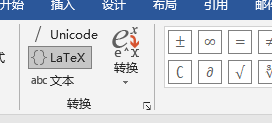如，当输入公式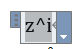点击转换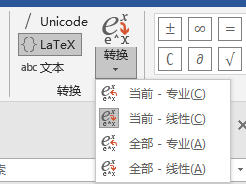可直接转换为公式 z i z^i

二、利用MatchType数学公式编辑软件
链接放下边，可以自取，在word中可直接在需要插入公式的位置插入LaTex代码

$数学公式$


然后选中公式，可以通过鼠标点击MathType命令栏中的Toggle Tex按钮，就会自动变成公式。也可以用快捷键Alt+\来完成，按一下变成公式，再按一次回到原来的样子。

MatchType链接：https://pan.baidu.com/s/1QX_ZapwwGDxN-MiUiE-Njg 提取码：74h3


## 常用特殊符号

LaTeX代码符号LaTeX代码符号
$\alpha$ α \alpha $\beta$ β \beta
$\gamma$ γ \gamma $\delta$ δ \delta
$\epsilon$ ϵ \epsilon $\theta$ θ \theta
$\Theta$ Θ \Theta $\partial$ ∂ \partial
$\lambda$ λ \lambda $\mu$ μ \mu
$\xi$ ξ \xi $\pi$ π \pi
$\rho$ ρ \rho $\sigma$ σ \sigma
$\tau$ τ \tau $\upsilon$ υ \upsilon
$\phi$ ϕ \phi $\chi$ χ \chi
$\psi$ ψ \psi $\omega$ ω \omega
$\carepsilon$ ε \varepsilon $\varphi$ φ \varphi
$\Omega$ Ω \Omega $\Psi$ Ψ \Psi
$\Phi$ Φ \Phi $\Sigma$ Σ \Sigma

## 上下标注导数向量等

名称latex公式符号
上标$a^2$ …… $a^{i+j}$ a 2 a^2 …… a i + j a^{i+j}
下标$a_2$ …… $a_{i+j} a 2 a_2 …… a i + j a_{i+j} 上下标$a^i_j$……$a^{i+1}_{j+1}$a j i a^i_j …… a j + 1 i + 1 a^{i+1}_{j+1} 前置上下标${}^2_1\!X^3_4$1 2 ⁣ X 4 3 {}^2_1\!X^3_4 导数$x’$……$\dot{x}$……$\ddot{x}$x ′ x' …… x ˙ \dot{x} …… x ¨ \ddot{x} 向量$\vec{x}$…$\overleftarrow{AB}$…$\overrightarrow{AB} … $\widehat{AB}$ x ⃗ \vec{x} A B ← \overleftarrow{AB} A B → \overrightarrow{AB} A B ^ \widehat{AB}
上划线$\overline{ABC}$ A B C ‾ \overline{ABC}
$\hat{a}$…… $\widehat{a}$ …… $\vec{a}$ a ^ \hat{a} …… a ^ \widehat{a} …… a ⃗ \vec{a}
上括号$\overbrace{1+2+\cdots+100}$ 1 + 2 + ⋯ + 100 ⏞ \overbrace{1+2+\cdots+100}
……$\begin{matrix}5050\\\overbrace{1+2+\cdots+100}\end{matrix}$ 5050 1 + 2 + ⋯ + 100 ⏞ \begin{matrix}5050\\\overbrace{1+2+\cdots+100}\end{matrix}
下括号$\underbrace{1+2+\cdots+100}$ 1 + 2 + ⋯ + 100 ⏟ \underbrace{1+2+\cdots+100}
$\begin{matrix}\underbrace{1+2+\cdots+100}\\5050\end{matrix}$ 1 + 2 + ⋯ + 100 ⏟ 5050 \begin{matrix}\underbrace{1+2+\cdots+100}\\5050\end{matrix}
求和$\sum_{i=1}^na_i$ …… $\sum\limits_{i=1}^na_i$ ∑ i = 1 n a i \sum_{i=1}^na_i …… ∑ i = 1 n a i \sum\limits_{i=1}^na_i
极限$\lim_{n\to\infty}x_n$ …… $\lim\limits_{n\to\infty}x_n$ lim ⁡ n → ∞ x n \lim_{n\to\infty}x_n …… lim ⁡ n → ∞ x n \lim\limits_{n\to\infty}x_n
积分$\int_{-N}^{N}e^x\,dx$ ∫ − N N e x   d x \int_{-N}^{N}e^x\,dx
双重积分$\iint_{-N}^{N}e^x\,dx\,dy$ ∬ − N N e x   d x   d y \iint_{-N}^{N}e^x\,dx\,dy
空格$a \quad b$ a b a \quad b

## 分数矩阵

名称latex公式符号
分数$\frac{1}{2+\frac{1}{2}}$ 1 2 + 1 2 \frac{1}{2+\frac{1}{2}}
二项式系数$\dbinom{n}{m}=\dbinom{n}{n-m}=C_n^m=C_n^{n-m}$ ( n m ) = ( n n − m ) = C n m = C n n − m \dbinom{n}{m}=\dbinom{n}{n-m}=C_n^m=C_n^{n-m}
矩阵$\begin{matrix}a&b\c&d\end{matrix}$ a b c d \begin{matrix}a&b\\c&d\end{matrix}
$\begin{vmatrix}a&b\\c&d\end{vmatrix}$ ∣ a b c d ∣ \begin{vmatrix}a&b\\c&d\end{vmatrix}
$\begin{bmatrix}a&\cdots&b\\\vdots&\ddots&\vdots\\c&\cdots&d\end{bmatrix}$ [ a ⋯ b ⋮ ⋱ ⋮ c ⋯ d ] \begin{bmatrix}a&\cdots&b\\\vdots&\ddots&\vdots\\c&\cdots&d\end{bmatrix}
$\begin{Bmatrix}a&c\\b&d\end{Bmatrix}$ { a c b d } \begin{Bmatrix}a&c\\b&d\end{Bmatrix}
$\begin{pmatrix}a&c\\b&d\end{pmatrix}$ ( a c b d ) \begin{pmatrix}a&c\\b&d\end{pmatrix}
分段函数$f(x)=\begin{cases}x-1&x\leqslant3\\x^2+3x-1&x>3\end{cases}$ f ( x ) = { x − 1 x ⩽ 3 x 2 + 3 x − 1 x > 3 f(x)=\begin{cases}x-1&x\leqslant3\\x^2+3x-1&x>3\end{cases}
方程组$\begin{cases}2x+9y-5z=10\\4x+20y+z=24\\x-\dfrac{1}{2}y+3z=8\end{cases}$ { 2 x + 9 y − 5 z = 10 4 x + 20 y + z = 24 x − 1 2 y + 3 z = 8 \begin{cases}2x+9y-5z=10\\4x+20y+z=24\\x-\dfrac{1}{2}y+3z=8\end{cases}

## 运算符

名称latex公式符号
根号$\surd$…$\sqrt{2}$ …$\sqrt[n]{}$ …$\sqrt[n]{x}$ √ \surd 2 \sqrt{2} n \sqrt[n]{} x n \sqrt[n]{x}
加减乘除$+ -$ …$\times$ … $\div$ …$pm$ + − + - × \times ÷ \div ± \pm
$\cdot$…$\bullet$ ⋅ \cdot ∙ \bullet
关系符$\ne$…$\sim$…$\approx$…$\leqslant$…$\geqslant$ ≠ \ne ∼ \sim ≈ \approx ⩽ \leqslant ⩾ \geqslant
箭头$\Rightarrow$……$\leftarrow$…$\Leftrightarrow$ ⇒ \Rightarrow …… ← \leftarrow ⇔ \Leftrightarrow
展开全文latex
• 按alt和+快捷键，即可进入公式输入框，可以使用latex语法写公式latex 公式
• 使用Word公式输入工具需要频繁地使用鼠标，因而编写公式会显得繁琐麻烦，那么有什么办法可以优雅地在Word中书写公式呢？其实Word在Word 2007就提供了像Latex一样使用代码书写公式的特性。详情可见Linear ...latex公式编号
• 1 引言 本人其习惯了写公式用LaTeX语法，Markdown的也是支持的。比如 J2(U)UJ1(U)=−K2(W)WK1(W) \frac{J_2...2 Mac os Word中插入LaTeX公式 （1）第一步：打开Mac下自带的软件Pages文稿。插入一个方程 （2）第二
• 键入ALT + “=”弹出公式编辑框，然后输入Latex公式，不过值得注意的是要将公式用{}框住，具体操作如下图： {FL\left(p_z^t\right)=-\alpha_z\left(1-p_z^t\right)^\gamma\log{\left(p_z^t\right)} 2.在word的功能...latex
• Mac 直接在word 使用latex编辑公式 直接选择word，插入公式 选择{Latex},开始书写公式，不熟练的话可以参考链接2，归类的很细致。 选择全部-专业，当输入较多的公式时可选择部分。选择线性则可进行编辑模式 示例...mac latex
• 本经验教授大家用Latex在word中编辑公式。 自行网上搜索安装包，安装MathType。（需要敲公式的学术狗，应该都装好了 文字嵌入公式需要插入公式的位置插入LaTex代码，例如∑i=19\sum_{i=1}^9∑i=19​, ...latex
•servlet java
• word中用Latex输入公式的小插件，可以代替mathtype，公式排版漂亮许多
• 文章目录mathtype上写latex公式word自动设置公式编号 没想到，学院这就开始组织开题报告了，所以不可避免地又要开始敲公式了，然而今时已不同往日，俺已经是个CSDN小博主啦，肯定不能继续用mathtype那种可视化...python
• 项目场景： 在word中原公式加入Latex语句 问题描述 原有公式上加入Latex...Word似乎不支持同一公式多次从latex字符转变为数学字符的操作 解决方案： 建议直接一次打完整个公式 若查到原因，后续会更新。 ...
• 1、打开word同时按住Alt+=，就会出现一个公式框，如下所示： 2、框内输入正常的LaTeX公式代码： 3、点击设计——转换——当前专业，将LaTeX代码转化为数学公式 4、成功啦~~
• 在word中，一种比较方便的做法是采用自带的公式（符号）插入的方式（如下图），但是这也需要大家不停地点击鼠标，如果公式量一大，也会稍微有一些崩溃。 这里也有一个简单的像LaTeX一样的方法能够键入公式。 ...python
• 本文推荐一款论文公式神器——Mathpix spin，它的功能是将你看到的公式截图翻译为LaTex格式，进而粘贴到如markdown等编辑器，转化为公式。再也不用担心输入公式啦。 1、Mathpix spin下载方式： 您可以直接去Math...
• 要点概览 目的doc文档插入latex代码以生成公式 ...但有时需要提交一些doc文档，需要用的编辑器是word或wps，这时如果要重新输入公式，那将会是很麻烦的一件事情，所以希望能重复利用在latex文档中输入latex 公式 aurora
• 公式转化为Word可识别模式 以\mathcal{G}为例，首先打开网址 http://engenharialivre.com/latex-para-word/?# 复制最下面方框的代码 <math xmlns="http://www.w3.org/1998/Math/MathML" display="block">...LaTeX
• 记得很久以前在word里打数学公式很痛苦，要用鼠标点啊点，效率奇低，包括像MathType那些工具。后来到了office 2007公式情况就不一样了，编辑器有了巨大的改进，适合我们喜欢用键盘的同学了。几乎所有的数学符号都...
• word输入LaTeX公式，插件有很多，前面使用的是一个叫做Aurora的插件，结果不是免费的，用了一段时间就要收费是，所以就不用了，从网上找到别人的介绍，可以使用TeXsword，下载使用了一下，还不错。 （1） ...
• 在Word中，遇到要输入带根号、导数、积分、各种格式的公式的时候，有没有想要砸电脑的冲动？5秒钟，就可以输入上面这样的公式的新技能要不要了解下？不需要安装复杂的软件，不需要记各种LateX的代码或快捷键，只需要...
• 首先打开word，然后输入latex代码，例如：我在word里输入 X^TX^{'} 选中它，然后插入里找到公式，找到Latex，点击Latex。 “转换”下面有个箭头，选择第一个。点击转换，你就会发现，你选中的Latex代码变成...latex
• 本文介绍通过Java程序在Word文档添加数学公式的方法。添加时，可添加latex数学公式或者MathML数学公式。详细内容见下文。 1. 程序环境 Word测试文档：.docx 2013 Word jar包：free spire.doc.jar 3.9.0 代码...Java latex
• 在Word中使用UnicodeMath和Latex优雅地输入线性公式在使用Word编写文章时，总会遇到书写数学公式的情况。使用Word公式输入工具需要频繁地使用鼠标，因而编写公式会显得繁琐麻烦，那么有什么办法可以优雅地在Word中...latex 公式
• latinmodern-math.otf 安装后即可在word中修改公式字体选择了 比自带的要好看的多
• 使用LATEX para Word网站：http://engenharialivre.com/latex-para-word/ 3、把MathML代码插入word公式框即可 word版本：Microsoft Office Professional Plus 2013 Mathpix版本：02.05.0009 ...latex
• 近期需要写一些课程论文，里面包含有不少的公式输入和排版上碰到了不少问题。也有不少博客介绍了表格法、制表符以及自动图文集，比如：Word 2016 撰写论文(1): 公式居中、编号右对齐。对比之下，当然我的这种方法......